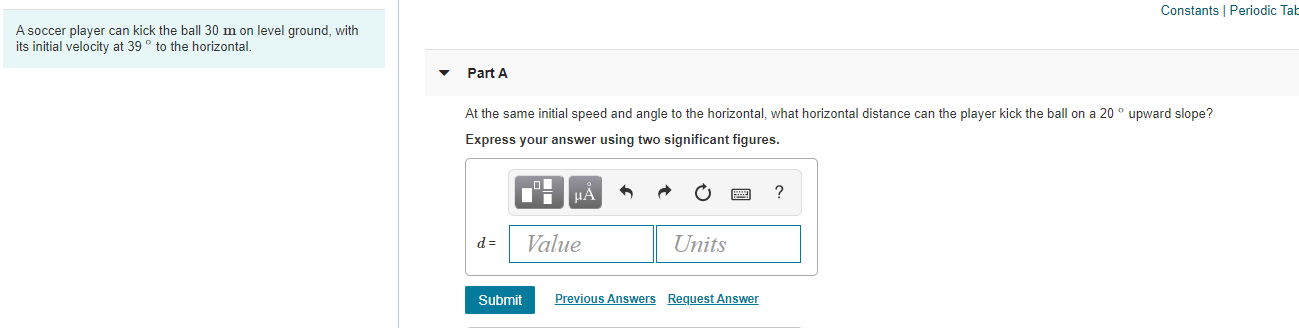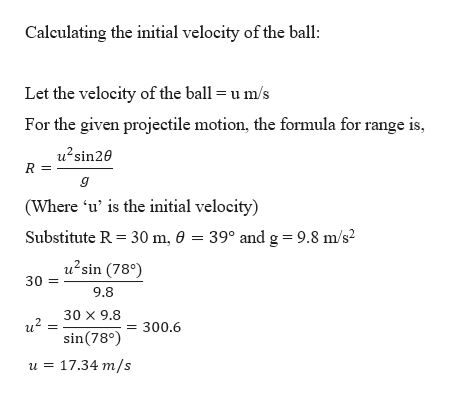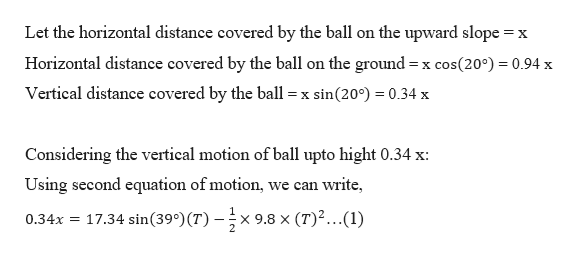Constants PeriodicA soccer player can kick the ball 30 m on level ground, withits initial velocity at 39 to the horizontal.Part AAt the same initial speed and angle to the horizontal, what horizontal distance can the player kick the ball on a 20upward slope?Express your answer using two significant figuresНАValueUnitsd =Previous AnswersRequest AnswerSubmit

Questionhelp_outlineImage TranscriptioncloseConstants Periodic A soccer player can kick the ball 30 m on level ground, with its initial velocity at 39 to the horizontal. Part A At the same initial speed and angle to the horizontal, what horizontal distance can the player kick the ball on a 20 upward slope? Express your answer using two significant figures НА Value Units d = Previous Answers Request Answer Submit fullscreen
Step 1

Given:

Horizontal range = 30 m

Angle of projection = 39 degrees

Step 2

Calculating the initial velocity of the ball:help_outlineImage TranscriptioncloseCaleulating the initial velocity of the ball Let the velocity of the ball= u m/s For the given projectile motion, the formula for range is, u2sin20 R (Where 'u' is the initial velocity) Substitute R 30 m, 0 = 39° and g = 9.8 m/s2 u2sin (78) 30 9.8 30 X 9.8 sin(78°300.6 u 17.34 m/s fullscreen
Step 3

Finding the horizontal distance covered by ...help_outlineImage TranscriptioncloseLet the horizontal distance covered by the ball on the upward slope x Horizontal distance covered by the ball on the ground x cos(200) 0.94 x Vertical distance covered by the ball sin(20) 0.34 x = X Considering the vertical motion of ball upto hight 0.34 x: can write Using second equation of motion we 17.34 sin(39°)(T)-;x x 9.8 x (T)...(1) 0.34x fullscreen

Want to see the full answer?

See Solution

Want to see this answer and more?

Our solutions are written by experts, many with advanced degrees, and available 24/7

See Solution
Tagged in

Kinematics